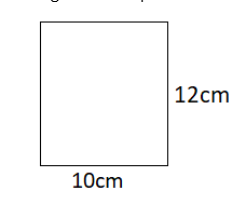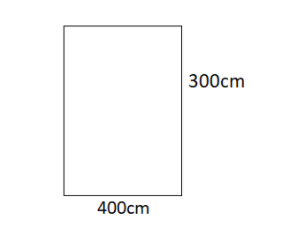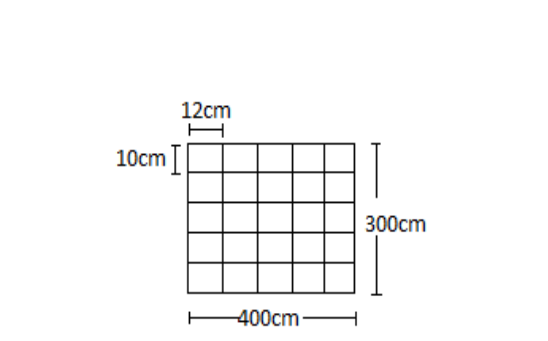Courses
Courses for Kids
Free study material
Offline Centres
MoreLast updated date: 01st Dec 2023
Total views: 280.2k
Views today: 2.80k

# A marble tile measures $10cm \times 12cm$ . How many tiles will be required to cover a wall of size $3m \times 4m$ ? Also, find the total cost of the tiles at the rate of $2$per tiles.Verified
280.2k+ views
Hint: In this problem, the number of required tiles will be the area of the rectangular region by the area of tiles. Thus find the area of the rectangular region and tiles separately. Then divide them to get the number of tiles that will fit in a rectangular region. Then, We have to convert the units (meter to cm). we know that $1m = 100cm$

In order to determine the number of tiles it will be required to cover a wall of size $3m \times 4m$ and also, find the total cost of the tiles at the rate of $2$ per tiles.
We are given marble tiles measuring $10cm \times 12cm$ .
Total area of marble tiles:
Let the area of marble tiles =Length × Breadth
We know that,
Length= $10cm$
Breadth= $12cm$
So, the area of marble tiles =Length × Breadth $= 10cm \times 12cm = 120c{m^2}$
Diagram for represent the marble tiles with the measurement:Therefore, We got the total area of marble tiles is $120c{m^2}$ --------- (1)
Now, we have to find the total cost of the tiles at the rate of $2$ per tiles.
The total area of wall:
Let the area of wall = Length × Breadth
We know that, $1meter = 100cm$
So,
Length $= 3m = 300cm$
Breadth $= 4m = 400cm$
Diagram for represent the area of wall with the measurement:Now, the area of wall = Length × Breadth $= 300 \times 400 = 120000$
Therefore, we found the total area of wall = $120000c{m^2}$ -------- (2)
Diagram for represent the wall:We use the formula for finding the required number of tiles,
Number of tiles required $= \dfrac{{Total{\text{ }}area{\text{ }}of{\text{ }}wall}}{{Total{\text{ }}area{\text{ }}of{\text{ }}marble{\text{ }}tile}}$ ------ (3)
By substituting all the value of equation (1) and (2) in the above formula, then
Number of tiles required= $\dfrac{{120000}}{{120}}$ = $1000$
We know that, The cost of the per tiles = $2$ Rs
So, The total cost of the tiles at the rate of $2$ per tiles = Number of tiles required× $2$ $= 1000 \times 2 = 2000Rs.$
Therefore, Total cost of tiles= $2000$ Rs
Hence, the total cost of the tiles at the rate of $2$per tiles is $= 1000 \times 2 = 2000Rs.$

Note: Area of rectangle is the region covered by the rectangle in a two-dimensional plane.